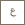#Top# Diogo Gomes

#### `Professor, Applied Mathematics and Computational Science Chair, Applied Mathematics and Computational Science Program`

Computer, Electrical and Mathematical Science and Engineering Division

## Education Profile

• 2006, Habilitation in Mathematics, Universidade Tecnica de Lisboa, Portugal
• 2000, Ph.D. in Mathematics, University of California at Berkeley, USA1996, M.Sc. in Mathematics, Instituto Superior Tecnico, Portugal1995, B.Sc. in Physics Engineering, Instituto Superior Tecnico, Portugal

## Research Interests

Professor Gomes’s research interests are in partial differential equations (PDE), namely on viscosity solutions of elliptic, parabolic and Hamilton-Jacobi equations as well as in related mean-field models. This area includes a large class of PDEs and examples, ranging from classical linear equations to highly nonlinear PDEs, including the Monge-Ampere equation, geometric equations for image processing, non-linear elasticity equations and the porous media equation. His research is motivated directly or indirectly by concrete applications. These include population and crowd modeling, price formation and extended mean-field models, numerical analysis of infinite dimensional PDEs and computer vision. Before joining KAUST, Professor Gomes has been a Professor in the Mathematics Department at the Instituto Superior Tecnico since 2001. He did his Post-Doc at the Institute for Advanced Study in Princeton in 2000 and at the University of Texas at Austin in 2001.

## Selected Publications

• Gomes, Diogo; Sánchez Morgado, Héctor (2014); A stochastic Evans-Aronsson problem. Trans. Amer. Math. Soc. 366 (2), 903–929.
• Cagnetti, F., Gomes, D., & Tran, H. V. (2013). Adjoint methods for obstacle problems and weakly coupled systems of PDE. ESAIM - Control, Optimisation and Calculus of Variations, 19(3), 754-779.
• Gomes, D. A., Mohr, J., & Souza, R. R. (2013). Continuous time finite state mean field games. Applied Mathematics and Optimization, 68 (1), 99-143.
• Cagnetti, F., Gomes, D., & Tran, H. V. (2011). Aubry-mather measures in the nonconvex setting. SIAM Journal on Mathematical Analysis, 43 (6), 2601-2629.
• Gomes, D. A., Mohr, J., & Souza, R. R. (2010). Discrete time, finite state space mean field games. Journal Des Mathematiques Pures Et Appliquees, 93(3), 308-328.